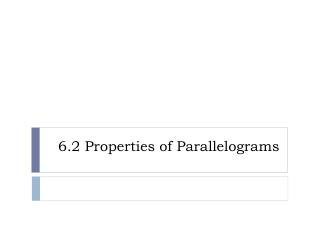DownloadDownload Presentation6.2 Properties of Parallelograms

# 6.2 Properties of Parallelograms

Download Presentation## 6.2 Properties of Parallelograms

- - - - - - - - - - - - - - - - - - - - - - - - - - - E N D - - - - - - - - - - - - - - - - - - - - - - - - - - -
##### Presentation Transcript

1. 6.2 Properties of Parallelograms

2. Q R P S Q R P S Q R P S Q R P S Theorem 6.2 If a quadrilateral is a parallelogram, then its opposite sides are congruent. PQ = RS and SP = QR Theorem 6.3 If a quadrilateral is a parallelogram, then it opposite angles are congruent. <P = < R and < Q = < S Theorem 6.4 If a quadrilateral is a parallelogram, then its consecutive angles are supplementary. m<P + m<Q = 180o, m<Q + m<R = 180o m<R + m<S = 180o, m<S + m<P = 180o Theorem 6.5 If a quadrilateral is a parallelogram, then its diagonals bisect each other. QM = SM and PM = RM _ _ _ _ ~ ~ ~ ~ _ _ _ _ ~ ~ Properties of Parallelograms

3. Theorems • 6.2 If a quadrilateral is a parallelogram, then its opposite sides are congruent. • 6.3 If a quadrilateral is a parallelogram, then its opposite angles are congruent. Q R S P

4. Theorems • 6.4 If a quadrilateral is a parallelogram, then its consecutive angles are supplementary. m<P + m<Q = 180° m<Q + m<R = 180° m<R + m<S = 180° m<S + m<P = 180° Q R S P

5. Using Properties of Parallelograms • PQRS is a parallelogram. Find the angle measure. • m< R • m< Q Q R 70 ° + m < Q = 180 ° m< Q = 110 ° 70° P S

6. 3 3 Using Algebra with Parallelograms • PQRS is a parallelogram. Find the value of h. 3h + 120 = 1800 - 120 -120 3h = 60 P Q h = 20 3h 120° S R

7. Theorems • 6.5 If a quadrilateral is a parallelogram, then its diagonals bisect each other. R Q M P S

8. Using properties of parallelograms • FGHJ is a parallelogram. Find the unknown length. • JH • JK 5 3 5 F G 3 K J H

9. Examples • Use the diagram of parallelogram JKLM. Complete the statement. LM K L NK <KJM N <LMJ NL MJ J M

10. Find the measure in parallelogram LMNQ. • LM • LP • LQ • QP • m<LMN • m<NQL • m<MNQ • m<LMQ 18 8 L M 9 110° 10 10 70° 9 P 8 70 ° 32° Q N 110 ° 18 32 °